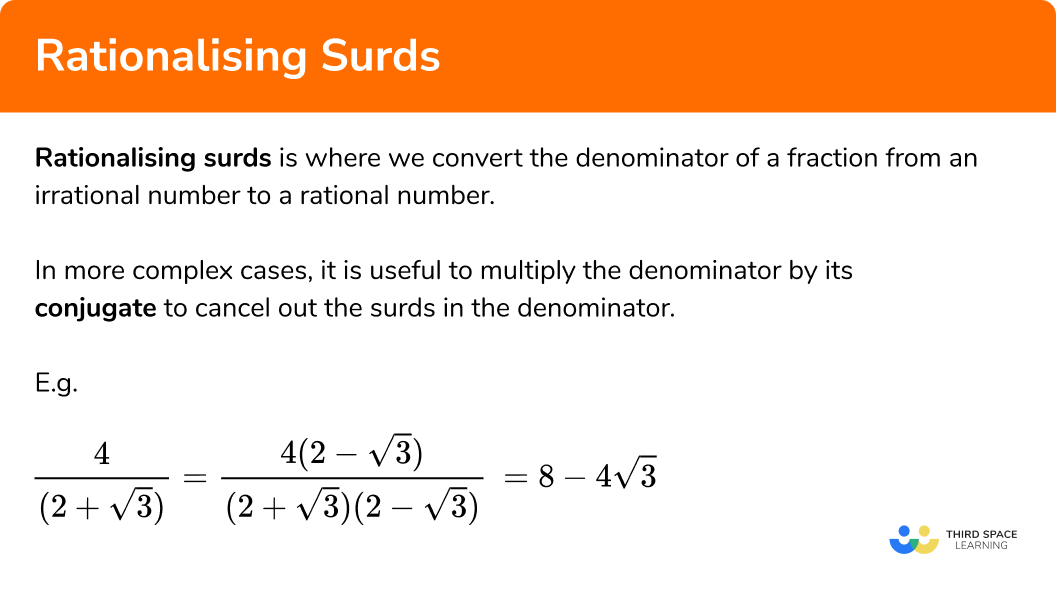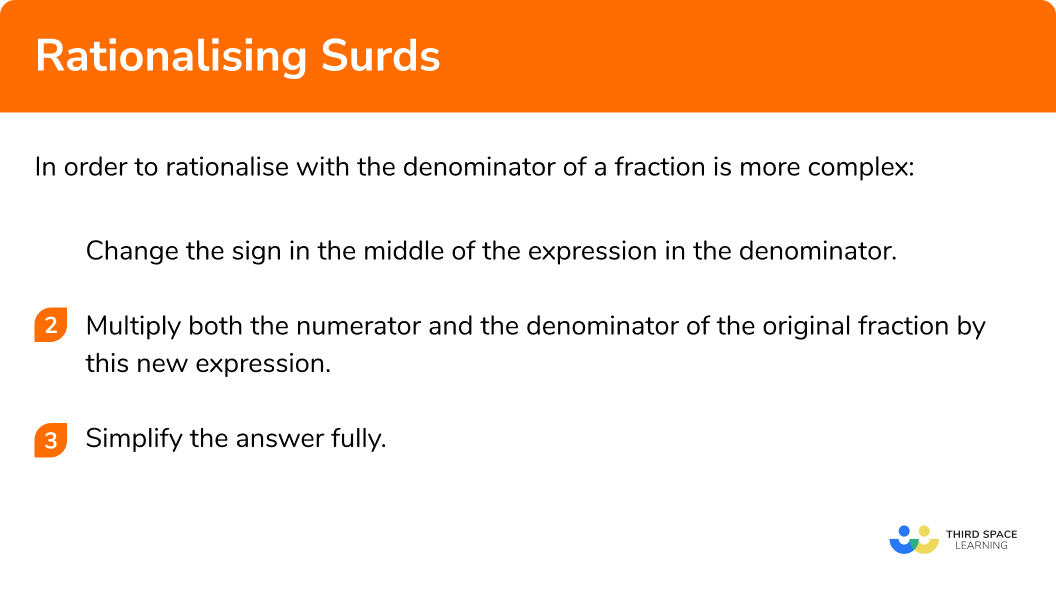GCSE Maths Number Surds

Rationalising Surds

# Rationalising Surds

Here we will learn about rationalising surds, including how to rationalise the denominator of more complicated surd expressions.

There are also rationalising denominators worksheets based on Edexcel, AQA and OCR exam questions, along with further guidance on where to go next if you’re still stuck.

## What is rationalising surds?

Rationalising surds is where we convert the denominator of a fraction from an irrational number to a rational number.

In the page Rationalising the Denominator we saw examples of how to rationalise simple surd denominators, by multiplying by the surd term.

E.g

\frac{6}{\sqrt{3}}=\frac{6\times \sqrt{3}}{\sqrt{3}\times \sqrt{3}}=\frac{6\sqrt{3}}{3}=2\sqrt{3}

However, we also saw that this method doesn’t work if the denominator is more complex.

In more complex cases, it is useful to multiply the denominator by its conjugate to cancel out the surds in the denominator.

E.g.

\frac{4}{(2+\sqrt{3})}=\frac{4(2-\sqrt{3})}{(2+\sqrt{3})(2-\sqrt{3})}=8-\sqrt{3}

This lesson looks at rationalising surd expressions with more complicated denominators. For simpler cases, where the denominator is a single surd, see the step-by-step guide for rationalising denominators.

Let’s look at an example:

E.g.

Rationalise the surd

\frac{4}{2+\sqrt{3}}

Simply multiplying by the square root of 3 won’t help, because we will still be left with a surd in the denominator, as demonstrated:

\frac{4 \times \sqrt{3}}{(2+\sqrt{3}) \times \sqrt{3}}=\frac{4 \sqrt{3}}{2 \sqrt{3}+3}

So instead we need to multiply the numerator and denominator by the conjugate of the denominator:

The conjugate of 2+\sqrt{3} is 2-\sqrt{3}

A conjugate or complementary expression contains the same numbers, but with the opposite sign in the middle.

When we multiply the denominator of the fraction by its conjugate the surd parts cancel each other out and we’re left with just an integer.

This is very similar to what happens when expanding a difference of two squares with quadratics (coming soon).

\begin{aligned} &(2+\sqrt{3})(2-\sqrt{3}) \\\\ &=4+2 \sqrt{3}-2 \sqrt{3}-3 \\\\ &=1 \end{aligned}

So to rationalise surd and simplify the expression we need to multiply the numerator and the denominator by the conjugate of the denominator.

\frac{4}{(2+\sqrt{3})}=\frac{4(2-\sqrt{3})}{(2+\sqrt{3})(2-\sqrt{3})}=\frac{8-4\sqrt{3}}{1}=8-4\sqrt{3}

### What is rationalising surds?## How to rationalise surds with more complex denominators

In order to rationalise when the denominator of a fraction is more complex:

1. Change the sign in the middle of the expression in the denominator.
2. Multiply both the numerator and the denominator of the original fraction by this new expression.

### How to rationalise surds with more complex denominators## Rationalising surds examples

### Example 1: using the conjugate of the denominator

Rationalise the denominator:

\frac{4}{2+\sqrt{3}}

1. Change the sign of the expression in the denominator.

The denominator is

(2+\sqrt{3})

so the conjugate is

(2-\sqrt{3})

2Multiply both the numerator and the denominator of the original fraction by this new expression.

\frac{4 \times(2-\sqrt{3})}{(2+\sqrt{3})(2-\sqrt{3})}

Numerator:

4 \times(2-\sqrt{3})=8-4 \sqrt{3}

Denominator:

\begin{aligned} (2+\sqrt{3})(2-\sqrt{3}) &=4+2 \sqrt{3}-2 \sqrt{3}-3 \\\\ &=4-3 \\\\ &=1 \end{aligned}

So the full expression becomes:

\frac{8-4 \sqrt{3}}{1}

The denominator is now rationalised, because 1 is a rational number.

1 is a factor of 8 and 4, so we can divide through by 1, leaving the answer as:

8-4 \sqrt{3}

### Example 2: using the conjugate of the denominator

Rationalise the denominator:

\frac{3 \sqrt{2}}{8-4 \sqrt{3}}

Change the sign of the expression in the denominator.

Multiply both the numerator and the denominator of the original fraction by this new expression.

### Example 3: binomial surd expressions on the top and bottom

Rationalise the denominator:

\frac{5-\sqrt{5}}{3+\sqrt{5}}

Change the sign of the expression in the denominator.

Multiply both the numerator and the denominator of the original fraction by this new expression.

### Common misconceptions

• Take care when expanding double brackets when using a conjugate surd expression

Remember to multiply everything in the first bracket by everything in the second, and check that you have four terms before simplifying.

• On step 3, if dividing integers to simplify, check that the factor you want to divide by is common to all of the integers in the numerator, as well as the denominator

E.g.

\frac{9+6 \sqrt{2}}{3}=3-2 \sqrt{2}

This can be simplified, because 3 is a factor of 3, 9 and 6. However,

\frac{4+6 \sqrt{2}}{3} cannot be simplified further, because 3 isn’t a factor of 4.

Rationalising surds is part of our series of lessons to support revision on surds. You may find it helpful to start with the main surds lesson for a summary of what to expect, or use the step by step guides below for further detail on individual topics. Other lessons in this series include:

### Practice rationalising surds questions

1. Rationalise the denominator

\frac{1}{4+\sqrt{3}}

4-\sqrt{3}\frac{4-\sqrt{3}}{13}\frac{4+\sqrt{3}}{4-\sqrt{3}}13Multiply the top and bottom by 4+\sqrt{3} to give:

\frac{1(4+\sqrt{3})}{(4-\sqrt{3})(4+\sqrt{3})}

The denominator simplifies to 16-3=13, and the numerator expands to become 4+\sqrt{3}.

2. Rationalise the denominator

\frac{5}{4-\sqrt{2}}

7+5 \sqrt{2}20-5 \sqrt{2}\frac{20+5 \sqrt{2}}{14}\frac{20+5 \sqrt{2}}{4}Multiply the top and bottom by 4+\sqrt{2} to give:

\frac{5(4+\sqrt{2})}{(4-\sqrt{2})(4+\sqrt{2})}

The denominator simplifies to 16-2=14 and the numerator expands to become 20+5 \sqrt{2}.

3. Rationalise the denominator

\frac{2 \sqrt{5}}{3-\sqrt{5}}

\frac{5+3 \sqrt{5}}{2}\frac{6 \sqrt{5}-10}{4}\frac{2}{3}\frac{5 \sqrt{5}}{4}Multiply the top and bottom by 3+\sqrt{5} to give:

\frac{2 \sqrt{5}(3+\sqrt{5})}{(3-\sqrt{5})(3+\sqrt{5})}

The denominator simplifies to 9-5=4, and the numerator expands to become 6 \sqrt{5}+10. Divide through by 2 to give the fully simplified final answer.

4. Rationalise the denominator

\frac{12-\sqrt{3}}{\sqrt{12}-3}

4 \sqrt{12}-\sqrt{3}+1010+7\sqrt{3}\frac{21\sqrt{3}+30}{9}\frac{12+\sqrt{3}}{3}Multiply the top and bottom by \sqrt{12}-3 to give:

\frac{(12-\sqrt{3})(\sqrt{12}+3)}{(\sqrt{12}-3)(\sqrt{12}+3)}

The denominator simplifies to 12-9=3 and the numerator expands to become 12 \sqrt{12}-3 \sqrt{3}-\sqrt{36}+36.

Simplify \sqrt{12}=2 \sqrt{3} and \sqrt{36}=6, then collect like terms. Finally, divide through by 3.

### Rationalising surds GCSE questions

1. Show that \frac{\sqrt{28}-8}{\sqrt{7}+2} can be written as 10-4\sqrt{7}.

(4 marks)

\sqrt{28}=2\sqrt{7}

(1)

\frac{(\sqrt{28}-8)(\sqrt{7}-2)}{(\sqrt{7}+2)(\sqrt{7}-2)}

(1)

\frac{30-12\sqrt{7}}{3}

(1)

= 10-4\sqrt{7} as required

(1)

2. Show that \frac{(\sqrt{27}+\sqrt{3})^{2}}{\sqrt{12}-3} can be written in the form a+b \sqrt{3}.

(4 marks)

\sqrt{12}=2 \sqrt{3}

(1)

(\sqrt{27}+\sqrt{3})^{2}=12

(1)

\frac{12(2\sqrt{3}+3)}{3}

(1)

=8 \sqrt{3}+12

(1)

## Learning checklist

You have now learned how to:

• Rationalise surds when the denominator contains a binomial

## Still stuck?

Prepare your KS4 students for maths GCSEs success with Third Space Learning. Weekly online one to one GCSE maths revision lessons delivered by expert maths tutors.

Find out more about our GCSE maths tuition programme.